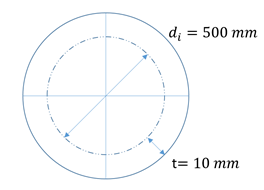## Tangential Stress

Consider an air cylinder’s pressure gauge reads 1,700 kPa. If the cylinder is made of a 10 mm steel rolled plate, with an internal diameter of 500 mm, what is the tangential stress inside the tank?Hint
If the cylinder is thin walled if
$$\frac{t}{\frac{d_{i}}{2}}\leq 0.10$$$where $$t$$ is the wall thickness, and $$d_i$$ is the inner diameter. Hint 2 The stress formula is: $$\sigma _{t}=\frac{P_{i}r}{t}$$$
First, we need to determine if the cylinder can be considered thin-walled, by verifying the below relationship:
$$\frac{t}{\frac{d_{i}}{2}}\leq 0.10\rightarrow \frac{10mm}{\frac{500mm}{2}}=0.04\leq 0.10\rightarrow cylinder\:is\:thin\:walled$$$Thus, the tangential stress formula is: $$\sigma _{t}=\frac{P_{i}r}{t}$$$
$$r=\frac{r_{i}+r_{o}}{2}=\frac{250mm+260mm}{2}=255mm$$$Finally, $$\sigma _{t}=\frac{P_{i}r}{t}=\frac{1.7MPa\cdot 255mm}{10mm}=43.35\:MPa$$$